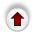## 2.2. Deleting a Value From a B-Tree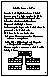Recall our deletion algorithm for binary search trees: if the value to be deleted is in a node having two subtrees, we would replace the value with the largest value in its left subtree and then delete the node in the left subtree that had contained the largest value (we are guaranteed that this node will be easy to delete).

We will use a similar strategy to delete a value from a B-tree. If the value to be deleted does not occur in a leaf, we replace it with the largest value in its left subtree and then proceed to delete that value from the node that originally contained it. For example, if we wished to delete 67 from the above tree, we would find the largest value in 67's left subtree, 66, replace 67 with 66, and then delete the occurrence of 66 in the left subtree. In a B-tree, the largest value in any value's left subtree is guaranteed to be in leaf. Therefore wherever the value to be deleted initially resides, the following deletion algorithm always begins at a leaf.

To delete value X from a B-tree, starting at a leaf node, there are 2 steps:

1. Remove X from the current node. Being a leaf node there are no subtrees to worry about.

2. Removing X might cause the node containing it to have too few values.

Recall that we require the root to have at least 1 value in it and all other nodes to have at least (M-1)/2 values in them. If the node has too few values, we say it has underflowed.

If underflow does not occur, then we are finished the deletion process. If it does occur, it must be fixed. The process for fixing a root is slightly different than the process for fixing the other nodes, and will be discussed afterwards.

How do we fix a non-root node that has underflowed? Let us take as a specific example, deleting 6 from this B-tree (of degree 5):
`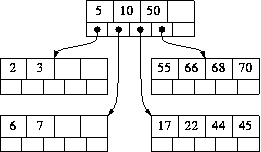`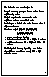Removing 6 causes the node it is in to underflow, as it now contains just 1 value (7). Our strategy for fixing this is to try to `borrow' values from a neighbouring node. We join together the current node and its more populous neighbour to form a `combined node' - and we must also include in the combined node the value in the parent node that is in between these two nodes.

In this example, we join node  with its more populous neighbour [17 22 44 45] and put `10' in between them, to create

[7 10 17 22 44 45]

How many values might there be in this combined node?

• The parent node contributes 1 value.
• The node that underflowed contributes exactly (M-1)/2-1 values.
• The neighbouring node contributes somewhere between (M-1)/2 and (M-1) values.
The treatment of the combined node is different depending on whether the neighbouring contributes exactly (M-1)/2 values or more than this number.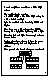Case 1: Suppose that the neighbouring node contains more than (M-1)/2 values. In this case, the total number of values in the combined node is strictly greater than 1 + ((M-1)/2 - 1) + ((M-1)/2), i.e. it is strictly greater than (M-1). So it must contain M values or more.

We split the combined node into three pieces: Left, Middle, and Right, where Middle is a single value in the very middle of the combined node. Because the combined node has M values or more, Left and Right are guaranteed to have (M-1)/2 values each, and therefore are legitimate nodes. We replace the value we borrowed from the parent with Middle and we use Left and Right as its two children. In this case the parent's size does not change, so we are completely finished.

This is what happens in our example of deleting 6 from the tree above. The combined node [7 10 17 22 44 45] contains more than 5 values, so we split it into:

• Left = [ 7 10 ]
• Middle = 17
• Right = [ 22 44 45 ]
Then put Middle into the parent node (in the position where the `10' had been) with Left and Right as its children (there is an error in this figure, it should not contain 6):
`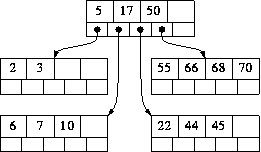`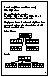Case 2: Suppose, on the other hand, that the neighbouring node contains exactly (M-1)/2 values. Then the total number of values in the combined node is 1 + ((M-1)/2 - 1) + ((M-1)/2) = (M-1)

In this case the combined node contains the right number of values to be treated as a node. So we make it into a node and remove from the parent node the value that has been incorporated into the new, combined node. As a concrete example of this case, suppose that, in the above tree, we had deleted 3 instead of 6. The node [2 3] underflows when 3 is removed. It would be combined with its more populous neighbour [6 7] and the intervening value from the parent (5) to create the combined node [2 5 6 7]. This contains 4 values, so it can be used without further processing. The result would be:

`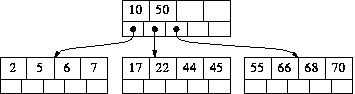`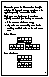It is very important to note that the parent node now has one fewer value. This might cause it to underflow - imagine that 5 had been the only value in the parent node. If the parent node underflows, it would be treated in exactly the same way - combined with its more populous neighbour etc. The underflow processing repeats at successive levels until no underflow occurs or until the root underflows (the processing for root-underflow is described next).

Now let us consider the root. For the root to underflow, it must have originally contained just one value, which now has been removed. If the root was also a leaf, then there is no problem: in this case the tree has become completely empty.

If the root is not a leaf, it must originally have had two subtrees (because it originally contained one value). How could it possibly underflow?

The deletion process always starts at a leaf and therefore the only way the root could have its value removed is through the Case 2 processing just described: the root's two children have been combined, along with the root's only value to form a single node. But if the root's two children are now a single node, then that node can be used as the new root, and the current root (which has underflowed) can simply be deleted.

To illustrate this, suppose we delete 7 from this B-tree (M=5):

`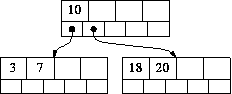`

The node [3 7] would underflow, and the combined node [3 10 18 20] would be created. This has 4 values, which is acceptable when M=5. So it would be kept as a node, and `10' would be removed from the parent node - the root. This is the only circumstance in which underflow can occur in a root that is not a leaf. The situation is this:

`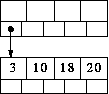`
Clearly, the current root node, now empty, can be deleted and its child used as the new root.